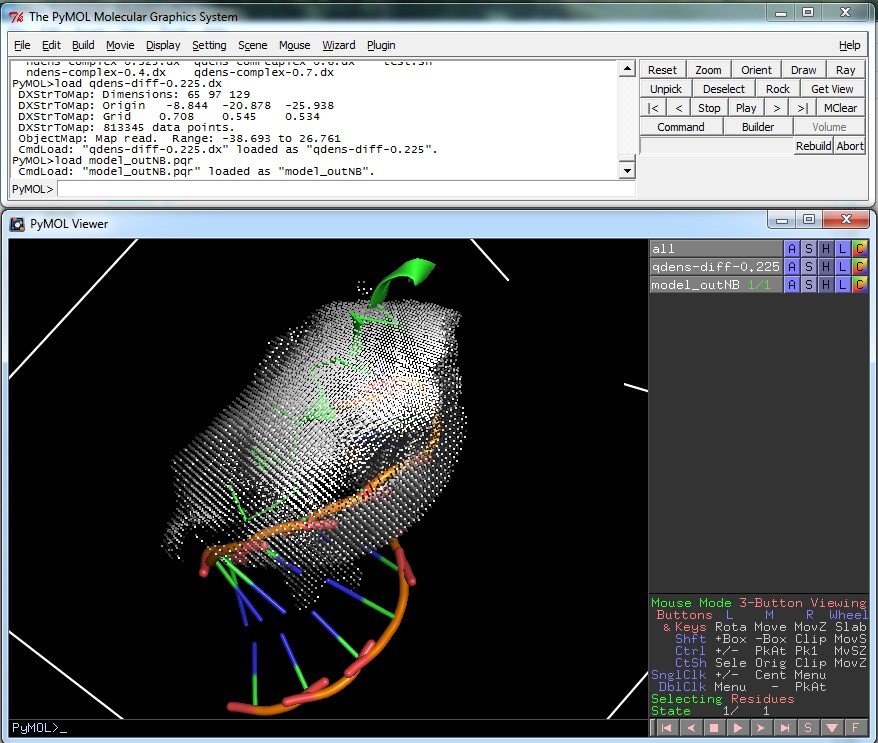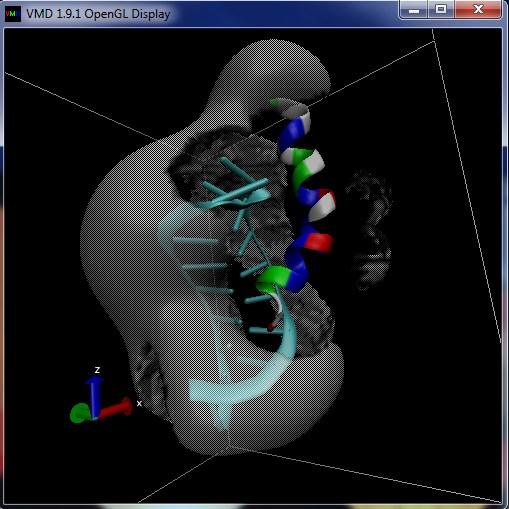Before reading this example, please review Caveats and sources of error for relevant caveats.

## Introduction¶

This example is taken from a paper by García-García and Draper. Special thanks to David Draper who provided the PDB files. This example explores the electrostatic contributions to the binding interaction between a 22-residue α-helical peptide of protein λ with the “box B” RNA hairpin structure. In particular, this example uses nonlinear Poisson-Boltzmann equation calculations to look at the non-specific screening effects of monovalent salt on the peptide-RNA complex. García-García and Draper isolated the contribution of KCl concentration to the binding of the folded peptide with the folded RNA hairpin and determined a fairly linear relationship between the binding free energy $$\Delta_{b} G$$ and the logarithm of the KCl concentration which yields

$\frac{\partial\Delta_{b}G}{\partial\log_{10}[{\rm KCl}]} = {6.0 \pm 0.2 ~ } {\rm kcal/mol}$

This slope can be used to determine the number of KCl ions linked to the binding equilibrium through the expression

$n = -\frac{\partial \Delta_b G}{{RT} \partial \log_{10}[{\rm KCl}]} = {-4.52 \pm 0.08~ } {\rm kcal/mol}$

where $$RT$$ is the thermal energy, to determine $$n = -4.4 \pm 0.2$$ for the RNA-peptide binding equilibrium. $$RT$$ is equal to $$kT * N_a$$ where $$kT$$ is the product of the Boltzmann constant $$k$$ (equal to the gas constant $$R/N_a$$), and the temperature $$T$$ (at STP it is 298.15 K) and $$N_a$$ is Avogadro’s constant. Thus $$RT$$ is equal to

$R ~ ({\mathrm{Joules}}/{\mathrm{Kelvin}}) * T~({\mathrm {Kelvin}}) * N_a~({\mathrm {mols}}) * {1~\mathrm{kJ}}/{1000~\mathrm J}$

which roughly equals

$(1.38 \times 10^{-23}) \times (6.022 \times 10^{23}) \times (298.15)/(1000)$

which is approximately 2.479 kJ/mol or 0.593 kcal/mol.

García-García and Draper used nonlinear Poisson-Boltzmann equation calculations to estimate the electrostatic contributions to the binding free energy as a function of the monovalent salt concentration. As discussed elsewhere, the Poisson-Boltzmann equation is only able to describe non-specific interactions of ions with solutes, including the effects of ion size and charge but otherwise ignoring the important differences between ionic species. Interestingly (and perhaps surprisingly), they find excellent agreement between the experimental binding energy dependence on KCl and their Poisson-Boltzmann calculations with equivalent concentrations of monovalent ions. This agreement strongly suggests that the binding of RNA and the peptide is primarily determined by electrostatic interactions. It also suggests that the primary interaction of the KCl with this system is through non-specific screening interactions. The García-García and Draper nonlinear Poisson-Boltzmann equation calculations gave:

$\frac{\partial\Delta_{b}G}{\partial\log_{10}[{\rm KCl}]} = {5.9 \pm 0.2 ~ } {\rm kcal/mol}$

and $$n = -4.3 \pm 0.2$$ for KCl linkage to the RNA-peptide binding equilibrium.

## APBS implementation¶

This example follows the calculations from their paper.

The PQR files are included in the examples/protein-rna/ directory of the apbs repository. This directory also includes a template.txt file that serves as a template for the APBS input files with IONSTR as a placeholder for the ionic strength. This file is also shown here:

read
mol pqr model_outNB.pqr
mol pqr model_outNpep.pqr
mol pqr model_outBoxB19.pqr
end
elec name complex
mg-auto
dime 65 97 129
cglen 45.3322 54.9498 82.2633
fglen 45.3322 52.3234 68.3902
cgcent mol 1
fgcent mol 1
mol 1
npbe
bcfl sdh
pdie 4.0
ion charge 1 conc IONSTR radius 2.0
ion charge -1 conc IONSTR radius 2.0
sdie 80.0
srfm mol
chgm spl2
sdens 10.00
swin 0.30
temp 298.15
calcenergy total
calcforce no
write qdens dx qdens-complex-IONSTR
write ndens dx ndens-complex-IONSTR
end
elec name peptide
mg-auto
dime 65 97 129
cglen 45.3322 54.9498 82.2633
fglen 45.3322 52.3234 68.3902
cgcent mol 1
fgcent mol 1
mol 2
npbe
bcfl sdh
pdie 4.0
sdie 80.0
ion charge 1 conc IONSTR radius 2.0
ion charge -1 conc IONSTR radius 2.0
srfm mol
chgm spl2
sdens 10.00
swin 0.30
temp 298.15
calcenergy total
calcforce no
write qdens dx qdens-peptide-IONSTR
write ndens dx ndens-peptide-IONSTR
end
elec name rna
mg-auto
dime 65 97 129
cglen 45.3322 54.9498 82.2633
fglen 45.3322 52.3234 68.3902
cgcent mol 1
fgcent mol 1
mol 3
npbe
bcfl sdh
pdie 4.0
sdie 80.0
ion charge 1 conc IONSTR radius 2.0
ion charge -1 conc IONSTR radius 2.0
srfm mol
chgm spl2
sdens 10.00
swin 0.30
temp 298.15
calcenergy total
calcforce no
write qdens dx qdens-rna-IONSTR
write ndens dx ndens-rna-IONSTR
end
print elecEnergy complex - peptide - rna end
quit


As used in the template file, the READ command, our calculation will have three parts:

• Calculation of the total electrostatic energy (including self-interaction energies) of the peptide-RNA complex. This calculation is named complex in the input file.
• Calculation of the total electrostatic energy (including self-interaction energies) of the peptide. This calculation is named peptide in the input file.
• Calculation of the total electrostatic energy (including self-interaction energies) of the RNA. This calculation is named rna in the input file.

The calculations themselves will not be overly demanding, since we will use relatively coarse grids. This grid coarseness has a significant impact on the absolute electrostatic binding energy we obtain from this particular calculation: the calculated energy isn’t converged with respect to grid spacing. However, the overall slope of binding energy with respect to monovalent ion concentration is rather insensitive with respect to the grid spacing, allowing us to save computational time and effort during the calculations. The calculation will conclude with a PRINT input file section command which will combine the total energies from the three parts to obtain our approximate absolute electrostatic binding energy for the complex at 0.225 M monovalent salt concentration. It is very important to note that this absolute energy no meaning in isolation for several reasons:

• It is not converged with respect to grid spacing
• It does not contain other very important non-electrostatic aspects of the binding energy which are important for the measured affinity

IONSTR is a placeholder that represents the ion concentration for the APBS calculation.

You will also have to create a dxmath.txt file which contains the following.

qdens-complex-IONSTR.dx
qdens-pep-IONSTR.dx -
qdens-rna-IONSTR.dx -
qdens-diff-IONSTR.dx =


dxmath will subtract the dx maps of the individual peptide and RNA from the overall structure (and prints to the qdens-diff-IONSTR.dx file.

## Automation with Python¶

We have provided Python scripts apbs_win, unix_dx.py that run the necessary APBS calculations and analyze the results. When you run these programs, you need to be in the same directory as template.txt and dxmath.txt. This script will create all the input files for the tests as well as run apbs and dxmath on your template.txt and dxmath.txt files. Most of the syntax fills in the ion concentrations in the template file, and the call commands actually run the calculations on each input.

## Visualization¶

The qdens-diff-0.225.dx file produced by the script can be viewed in PyMOL or another visualization program to give something similar to the following imaged which show the difference in charge density before and after binding.